Students can Download Samacheer Kalvi 10th Maths Model Question Paper 1 English Medium Pdf, Samacheer Kalvi 10th Maths Model Question Papers helps you to revise the complete Tamilnadu State Board New Syllabus and score more marks in your examinations.

## Tamilnadu Samacheer Kalvi 10th Maths Model Question Paper 1 English Medium

Instructions

• The question paper comprises of four parts.
• You are to attempt all the parts. An internal choice of questions is provided wherever applicable.
• All questions of Part I, II, III and IV are to be attempted separately.
• Question numbers 1 to 14 in Part I are Multiple Choice Quèstions of one-mark each. These are to be answered by choosing the most suitable answer from the given four alternatives and.writing the option code and the corresponding answer.
• Question numbers 15 to 28 in Part II àre two-marks questions. These are to be answered in about one or two sentences.
• Question numbers 29 to 42 in Part III are five-marks questions. These are to be answered in about three to five short sentences.
• Question numbers 43 to 44 in Part IV are eight-marks questions. These are to be answered in detail. Draw diagrams wherever necessary.

Time: 3 Hours
Max Marks: 100

PART – I

I. Choose the correct answer. Answer all the questions. [14 × 1 = 14]

Question 1.
If {(a, 8),(6, b)} represents an identity function, then the value of a and b are respectively ……………. .
(1) (8,6)
(2) (8,8)
(3) (6,8)
(4) (6,6) .
(1) (8,6)Question 2.
If the H.C.F of 65 and 117 is expressible in the form of 65m -117, then the value of m is ……………. .
(1) 4
(2) 2
(3) 1
(4) 3
(2) 2

Question 3.
The next term ol the sequence $$\frac{3}{16}, \frac{1}{8}, \frac{1}{12}, \frac{1}{18}$$ is …………… .
(1) $$\frac{1}{24}$$
(2) $$\frac{1}{27}$$
(3) $$\frac{2}{3}$$
(4) $$\frac{1}{81}$$
(2) $$\frac{1}{27}$$

Question 4.
Which of the following should be added to make x4 + 64 a perfect square ……………… .
(1) 4x2
(2) 16x2
(3) 8x2
(4) -8x2
(2) 16x2

Question 5.
Find the matrix x if 2x + $$\left[ \begin{matrix} 1 & 3 \\ 5 & 7 \end{matrix} \right] =\left[ \begin{matrix} 5 & 7 \\ 9 & 5 \end{matrix} \right]$$ ……………. .
(1) $$\left[ \begin{matrix} -2 & -2 \\ 2 & -1 \end{matrix} \right]$$
(2) $$\left[ \begin{matrix} 2 & 2 \\ 2 & -1 \end{matrix} \right]$$
(3) $$\left[ \begin{matrix} 1 & 2 \\ 2 & 2 \end{matrix} \right]$$
(4) $$\left[ \begin{matrix} 2 & 1 \\ 2 & 2 \end{matrix} \right]$$
(2) $$\left[ \begin{matrix} 2 & 2 \\ 2 & -1 \end{matrix} \right]$$

Question 6.
Two poles of heights 6 m and 11 m stand vertically on a plane ground if the distance between their feet is 12m, what is the distance between their tops?
(1) 13 m
(2) 14 m
(3) 15 m
(4) 12.8 m
(1) 13 m

Question 7.
If (5, 7), (3,p) and (6, 6) are collinear then the value of p is ……………… .
(1) 3
(2) 6
(3) 9
(4) 12
(3) 9

Question 8.
The value of sin2θ + $$\frac{1}{1+\tan ^{2} \theta}$$ is equal to …………….. .
(1) tan2θ
(2) 1
(3) cot2θ
(4) 0
(2) 1

Question 9.
If the radius of the base of a cone is tripled and the height is doubled then the volume is ……………… .
(4) unchangedQuestion 10.
Standard deviation of a collection of data is 2√2. If each value is multiplied by 3, then the standard deviation of the new data is
……………… .
(1) √l2
(2) 4√2
(3) 6√2
(4) 9√2
(3) 6√2

Question 11.
A page is selected at random from a book. The probability that the digit at unit place of the page number chosen’ is less than 7 is …………. .
(1) $$\frac{3}{10}$$
(2) $$\frac{7}{10}$$
(3) $$\frac{3}{9}$$
(4) $$\frac{7}{9}$$
(2) $$\frac{7}{10}$$

Question 12.
If f = {(6, 3), (8, 9), (5, 3), (-1, 6)} then the pre-images of 3 are ……………… .
(1) 5 and -1
(2) 6 and 8
(3) 8 and -1
(4) 6 and 5
(4) 6 and 5

Question 13.
If a and p are the zeros 6f the polynomial P(x) = 4x2 + 3x + 7 then $$\frac{1}{\alpha}+\frac{1}{\beta}$$ is equal to …………….. .
(1) $$\frac{7}{3}$$
(2) $$\frac{-7}{3}$$
(3) $$\frac{3}{7}$$
(4) $$\frac{-3}{7}$$
(4) $$\frac{-3}{7}$$

Question 14.
The probability that a-leap year will have 53 Fridays or 53 Saturdays is …………….. .
(1) $$\frac{2}{7}$$
(2) $$\frac{1}{7}$$
(3) $$\frac{4}{7}$$
(4) $$\frac{3}{7}$$
(4) $$\frac{3}{7}$$

PART – II

II. Answer any ten questions. Question No. 28 is compulsory. [10 × 2 = 20]

Question 15.
A relation “f” is defined by f(x) = x2 – 2 where x ∈ {-2, -1, 0, 3}
(i) List the elements of f
(ii) If f a function?
f(x) = x2 – 2 where x ∈ {-2,-1, 0, 3}
(i) f(-2) = (-2)2 – 2 = 2 ; f(-1) = (-1)2 – 2 = -1
f(0) = (0)2 – 2 = -2 ; f(3) = (3)2 – 2 = 7
Therefore, f = {(-2,2), (-1,-1), (0,-2), (3,7)}

(ii) We note that each element in the domain of f has a unique image.
Therefore f is a function.Question 16.
Let f(x) = x2 – 1. Find fofof
fofof = fof [(x)]
= fof (x2 – 1)
= f (x2 – 1)2 – 1
= f (x4 – 2x2 + 1 – 1)
= f (x4 – 2x2)
fofof = (x4 – 2x2)2 – 1

Question 17.
What is the smallest number that when divided by three numbers such as 35, 56 and 91 leaves remainder 7 in each case?
Find the L.C.M of 35, 56, and 91
35 = 5 × 7
56 = 2 × 2 × 2 × 7
91 = 7 × 13
L.C.M = 23 × 5 × 7 × 13
= 3640
Since it leaves remainder 7
The required number = 3640 + 7 = 3647
∴ The smallest number is 3647

Question 18.
In a G.P. 729, 243, 81,… find t7.
The G.P. is 729,243,81, …………..
a = 729; r = $$\frac{t_{2}}{t_{1}}=\frac{243}{729}=\frac{81}{243}=\frac{27}{81}=\frac{3}{9}=\frac{1}{3}$$
tn = a rn – 1
t7 = 729 $$\left(\frac{1}{3}\right)^{7-1}$$
= $$729\left(\frac{1}{3}\right)^{6}=729 \times \frac{1}{729}=1$$
∴ The 7th term is 1

Question 19.
Find the excluded values of the expression $$\frac{t}{t^{2}-5 t+6}$$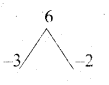The expression $$\frac{t}{t^{2}-5 t+6}$$ is undefined
when t2 – 5t + 6 = 0
(t – 3) (t – 2) = 0
t – 3 = 0 or t – 2 = 0
t = 3 or t = 2
The excluded values are 2 and 3

Question 20.
Find the square root of 9x2 – 24xy + 30xz – 40yz + 25z2 + 16y2
$$\sqrt{9 x^{2}-24 x y+30 x z-40 y z+25 z^{2}+16 y^{2}}$$
$$\sqrt{(3 x)^{2}+(4 y)^{2}+(5 z)^{2}-2(3 x)(4 y)-2(4 y)(5 z)+2(3 x)(5 z)}$$
$$\sqrt{(3 x-4 y+5 z)^{2}}$$ [using (a – b + c)2 = a2 + b2 + c2 -2ab -2bc + 2ac]
= |3x – 4y + 5z|

Question 21.
construct a 3 x 3 matrix whose elements are given by aij = $$\frac{(i+j)^{3}}{3}$$
aij = $$\frac{(i+j)^{3}}{3}$$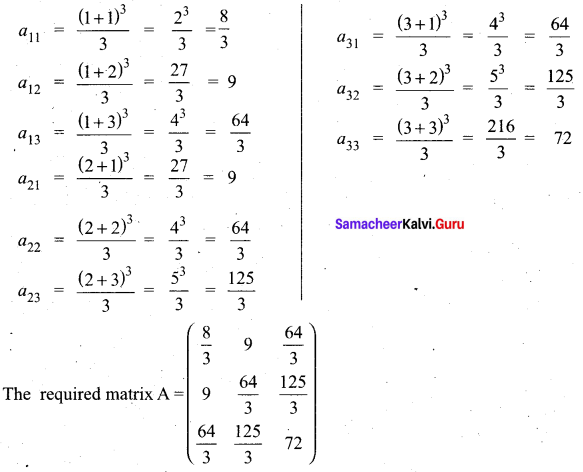Question 22.
If ∆ABC ~ ∆DEF such that area of ∆ABC is 9cm2 and the area of ∆DEF is 16 cm2 and BC = 2.1 cm. Find the length of EF.
Given ∆ABC ~ ∆DEF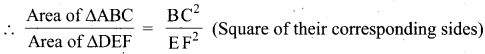$$\frac{9}{16}=\frac{(2.1)^{2}}{\mathrm{EF}^{2}}$$
$$\left(\frac{3}{4}\right)^{2}=\left(\frac{2.1}{\mathrm{EF}}\right)^{2}$$
$$\frac{3}{4}=\frac{2.1}{\mathrm{EF}}$$
EF = $$\frac{4 \times 2.1}{3}$$ = 2.8 cm
Length of EF = 2.8 cm

Question 23.
If the area of the triangle formed by the vertices (p,p), (5,6), (5, -2) is 32 sq.units. Find the value of ‘p’.
Let the vertices be A (p,p), B (5, 6) and C (5, -2)
Area of a triangle = 32 sq. units
$$\frac{1}{2}$$[x1y2 + x2y3 + x3y1) – (x2y1 + x3y2 + x1y3] = 32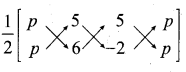$$\frac{1}{2}$$[(6p – 10 + 5p) – (5p + 30 – 2p)] = 32
$$\frac{1}{2}$$[11p – 10 – 3p – 30] = 32
11p – 10 – 3p – 30 = 64
8p – 40 = 64
8p = 64 + 40 ⇒ 8p = 104
p = $$\frac{104}{8}$$ = 13
The value of p = 13

Question 24.
Find the angle of elevation of the top of a tower from a point on the ground, which is 30 m away from the foot of a tower of height 10 √3 m.
Height of the tower (AC) = 10 √3 m
Distance between the base of the tower and point of observation (AB) = 30 m
Let the angle of elevation ∠ABC be θ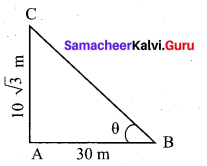In the right ∆ ABC, tan θ = $$\frac{A C}{A B}$$
$$=\frac{10 \sqrt{3}}{30}=\frac{\sqrt{3}}{3}$$
tan θ = $$\frac{1}{\sqrt{3}}$$ = tan 30°
∴ Angle of inclination is 30°

Question 25.
Find the diameter of a sphere whose surface area is 154m2.
Let r be the radius of the sphere.
Given that, surface area of sphere = 154 m2
4πr2 = 154
4 × $$\frac{22}{7}$$ × r2 = 154
gives r2 = $$154 \times \frac{1}{4} \times \frac{7}{22}$$
hence, r2 = $$\frac{49}{4}$$ we get r = $$\frac{7}{2}$$
Therefore, diameter is 7mQuestion 26.
Find the standard deviation and the variance of first 23 natural numbers.
Standard deviation of first “n” natural numbers = $$\sqrt{\frac{n^{2}-1}{12}}$$
Standard deviation of first 23 natural numbers = $$\sqrt{\frac{23^{2}-1}{12}}$$
= $$\sqrt{\frac{529 – 1}{12}}$$
$$=\sqrt{\frac{528}{12}}$$
= √44 (variance)
Variance = 44 and standard deviation = 6.63

Question 27.
Form the quadratic equation whose roots are 3 + √7 ; 3 – √7
Sum of the roots = 3 + √7 + 3 – √7
= 6
Product of the roots = (3 + √7)(3 – √7)
= 9 – 7 = 2 .
The required equation is
x2 – (sum of the roots) x + product of the roots = 0
x2 – (6) x + 2 = 0
x2 – 6x + 2 = 0

Question 28.
Two coins are tossed together. What is the probability of getting at most one head?
Sample space = {HH, HT, TH, TT}
n(S) = 4
Let A be the event of getting atmost one head A = {HT, TH, TT}
n(A) = 3
P(A) = $$\frac{n(\mathrm{A})}{n(\mathrm{S})}=\frac{3}{4}$$
The probability is $$\frac{3}{4}$$PART – III

III. Answer any ten questions. Question No 42 is compulsory. [10 × 5 = 50]

Question 29.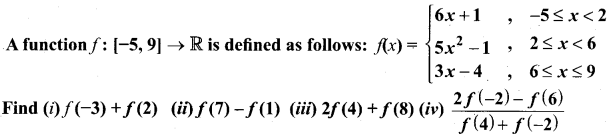f(x) = 6x + 1 ; x = {-5, -4, -3, -2, -1, 0, 1}
f(x) = 5x2 – 1 ; x = {2,3,4,5}
f(x) = 3x – 4; x = {6,7,8,9}

(i) f(-3) + f(2)
f(x) = 6x+1
f(3) = 6(-3) + 1 = -18 + 1 = -17
f(x) = 5x2 – 1
f(2) = 5(2)2 – 1 = 20 – 1 = 19
f(-3) + f(2) = -17 + 19 = 2

(ii) f(7) – f(1)
f(x) = 3x – 4
f(7) = 3(7) – 4= 21 – 4 = 17
f(x) = 6x + 1
f(1) = 6(1)+ 1 = 6 + 1 = 7
f(7) – f(1) = 17 – 7
= 10

(iii) 2f(4) + f(8)
f(x) = 5x2 – 1
f(4) = 5(4)2 – 1 = 15(16) – 1
= 80 – 1 = 79
f(x) = 3x – 4
f(8) = 3(8) – 4 = 24 – 4 = 20
2f(4) + f(8) = 2(79) + 20
= 158 + 20 .
= 178

(iv) $$\frac{2 f(-2)-f(6)}{f(4)+f(-2)}$$
f(x) = 6x + 1
f(-2) = 6(-2) + 1 = -12 + 1 = -11
f(x) = 3x – 4
f(6) = 3(6) – 4 = 18 – 4 = 14
f(x) = 5x2 – 1
f(4) = 5(4)2 – 1 = 5(16) – 1
= 80 – 1 = 79
f(x) = 6x+1
f(-2) = 6(-2) + 1 = -12 + 1 = -11
$$\frac{2 f(-2)-f(6)}{f(4)+f(-2)}$$ = $$\frac{2(-11)-14}{79-11}$$
= $$\frac{-22-14}{68}=\frac{-36}{68}=\frac{-9}{17}$$Question 30.
If f(x) = 2x + 3, g(x) = 1 – 2x and h(x) = 3x Prove that fo(goh) = (fog)oh
f(x) = 2x + 3, g(x) = 1 – 2x, h(x) = 3x
Now, (fog)(x) = f(g(x)) = f(1 – 2x) = 2(1 – 2x) + 3 = 5 – 4x
Then, (fog)oh(x) = (fog) (h(x)) = (fog)(3x) = 5 – 4(3x) = 5 – 12x ….(1)
(goh)(x) = g(h(x)) = g(3x) = 1 – 2(3x) = 1 – 6x
So, fo (goh)(x) = f(1 – 6x) = 2(1 – 6x) + 3 = 5 – 12x …….. (2)
From (1) and (2), we get (fog)oh = fo(goh)

Question 31.
If S1 , S2 , S3, ……. Smare the sums of n terms of m A.P.,s whose first terms are 1,2, 3, …….. m and whose common differences are 1,3,5,….(2m – 1) respectively, then show that (S1 + S2 + S3 + …….. + Sm) = $$\frac { 1 }{ 2 }$$ mn(mn + 1)
First terms of an A.P and 2, 3 ……….. m
The common difference are 1, 3, 5 , ………….. (2m – 1)
Sn = $$\frac { n }{ 2 }$$[2a+(n – 1)d]
S1 = $$\frac { n }{ 2 }$$[2+(n – 1)(1)]
= $$\frac { n }{ 2 }$$[2 + n – 1]
S1 = $$\frac { n }{ 2 }$$[n + 1] …….. (1)
S2 = $$\frac { n }{ 2 }$$[2(2) + (n – 1)3]
= $$\frac { n }{ 2 }$$[4 + 3n – 3]
S2 = $$\frac { n }{ 2 }$$(3n – 2) ……. (2)
S3 = $$\frac { n }{ 2 }$$[2(3) + (n – 1)5]
= $$\frac { n }{ 2 }$$[6 + 5n – 5}
= $$\frac { n }{ 2 }$$[5n + 1] …….. (3)
Sm = $$\frac { n }{ 2 }$$[2m + (n – 1)(2m – 1)]
= $$\frac { n }{ 2 }$$[2m + 2mn – n – m – 2m + 1]
= $$\frac { n }{ 2 }$$[n(2m – 1) + 1]
By adding (1) (2) (3) we get
S1 + S2 + S3 + ……….. + Sm = $$\frac { n }{ 2 }$$(n + 1) + $$\frac { n }{ 2 }$$(3n + 1) + $$\frac { n }{ 2 }$$(5n + 1)+ …………+ $$\frac { n }{ 2 }$$[n(2m – 1) + 1]
= $$\frac { n }{ 2 }$$[n + 1 + 3n + 1 + 5n + 1 …………… + n(2m – 1) + m]
= $$\frac { n }{ 2 }$$[n + 3n + 5n + ………….. + n(2m – 1) + m]
= $$\frac { n }{ 2 }$$[n$$(\frac { m }{ 2 })$$(2m) + m]
= $$\frac { n }{ 2 }$$[nm2 + m]
S1 + S2 + S3 + ……… + Sm = $$\frac { mn }{ 2 }$$[nm + 1]

Hint:
1 + 3 + 5 + …….. + 2m – 1
Sn = $$\frac { n }{ 2 }$$(a + 1)
= $$\frac { m }{ 2 }$$ (1 + 2m – 1)
= $$\frac { m }{ 2 }$$ (2m)Question 32.
Find the sum to n terms of the series 0.4 + 0.44 + 0.444 + ………. to n terms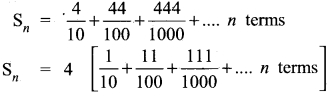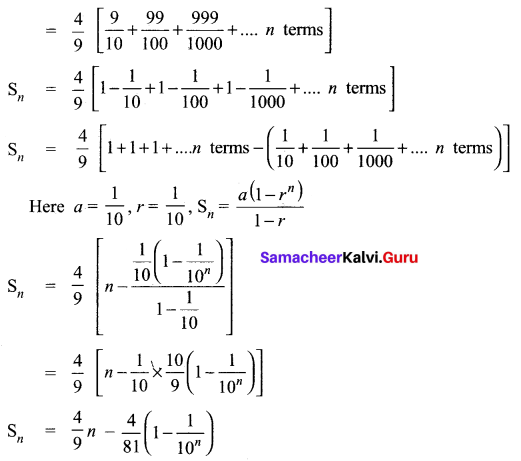Question 33.
Vani, her father and her grand father have an average age of 53. One-half of her grand father’s age plus one-third of her father’s age plus one fourth of Vani’s age is 65. If 4 years ago Vani’s grandfather was four times as old as Vani then how old are they all now?
Let the age of Vani be”x” years
Vani father age = “y” years
Vani grand father = “z” years .
By the given first condition.
$$\frac{x+y+z}{3}=53$$
x + y + z = 159 ….(1)
By the given 2nd condition.
$$\frac{1}{2} z+\frac{1}{3} y+\frac{1}{4} x=65$$
Multiply by 12
6z + 4y + 3x = 780 .
3x + 4y + 6z = 780 ….(2)
By the given 3rd condition
z – 4 = 4 (x – 4) ⇒ z – 4 = 4x – 16
– 4x + z = – 16 + 4
4x – z = 12 ….(3)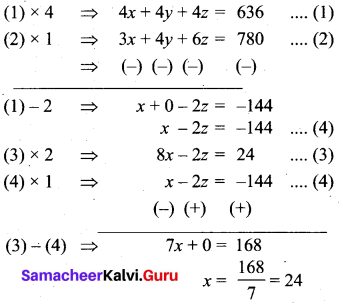Substitute the value of x = 24 in (3)
4 (24) – z = 12
96 – z = 12
– z = 12 – 96
z = 84

Substitute the value of x = 24 and
z = 84 in (1)
24 + y + 84 = 159
y + 108 = 159
y = 159 – 108
= 51

Vani age = 24 years
Vani’s father age = 51 years
Vani grand father age = 84 yearsQuestion 34.
Find the values of a and b if the following polynomials are perfect squares. ax4 + bx3 + 361x2 + 220.x + 100
Re-arrange the order we get .
100 + 220x + 361x2 + by3 + ax4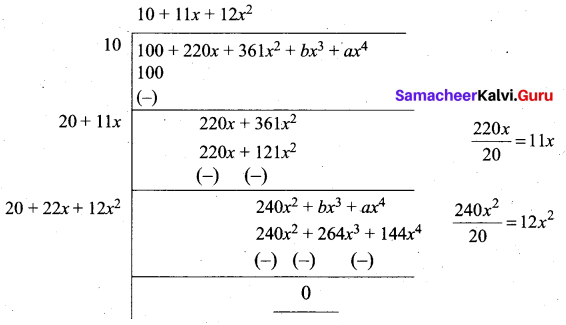Since it is a perfect square
b – 264 = 0
b = 264

a – 144 = 0
a = 144

∴ The value of a = 144 and b = 264

Question 35.
State and prove Pythagoras Theorem
Statement
In a right angle triangle, the square on the hypotenuse is equal to the sum of the squares on the other two sides.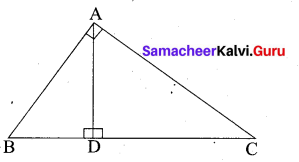Proof
Given : In ∆ABC, ∠A = 90°.
To prove : AB2 + AC2 = BC2
Construction : Draw AD ⊥ BC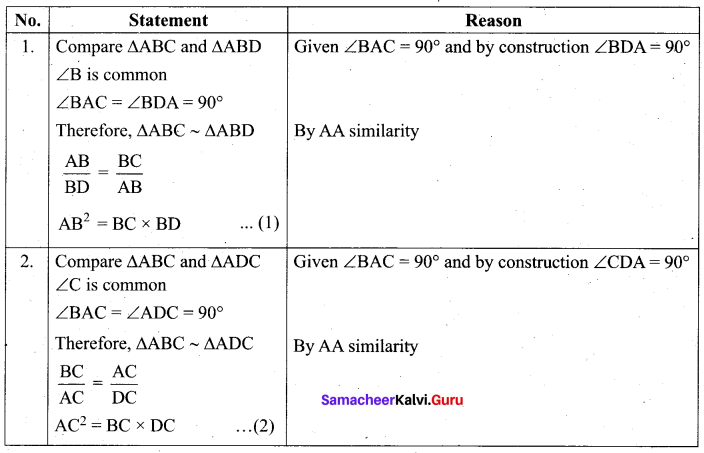Adding (1) and (2) we get
AB2 + AC2 = BC × BD + BC × DC
= BC (BD + DC) = BC × BC
AB2 + AC2 = BC2
Hence the theorem is proved.Question 36.
Find the equation of the median and altitude of AABC through A where the vertices are A(6, 2), B(-5, -1) and C(1,9).
(i) To find median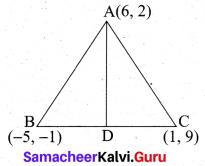Mid point of BC(D) = $$\left(\frac{x_{1}+x_{2}}{2}, \frac{y_{1}+y_{2}}{2}\right)$$
$$=\left(\frac{-5+1}{2}, \frac{-1+9}{2}\right)$$
$$=\left(\frac{-4}{2}, \frac{8}{2}\right)$$ = (-2, 4)
Equation of the median AD is
$$\frac{y-y_{1}}{y_{2}-y_{1}}=\frac{x-x_{1}}{x_{2}-x_{1}}$$
$$\frac{y-2}{4-2}=\frac{x-6}{-2-6}$$
$$\frac{y-2}{2}=\frac{x-6}{-8}$$
= 2(x – 6) = 8(y – 2)
2x – 12 = -8y + 16
2x + 8y – 28 = 0
(÷by 2) x + 4y – 14 = 0

(ii) To find the equation of the altitude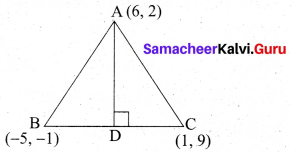Slope of BC = $$\frac{y_{2}-y_{1}}{x_{2}-x_{1}}$$
$$=\frac{9+1}{1+5}$$
$$=\frac{10}{6}=\frac{5}{3}$$
Slope of the altitude = $$-\frac{3}{5}$$
Equation of the altitude AD is
y – y1 = m(x – x1)
y – 2 = $$-\frac{3}{5}$$(x – 6)
-3 (x – 6) = 5 (y – 2)
-3x + 18 = 5y – 10
-3x – 5y + 18 + 10 = 0
-3x – 5y + 28 =0
3x + 5y – 28 = 0
∴ Equation of the median is x + 4y – 14 = 0
Equation of the altitude is 3x + 57 – 28 = 0Question 37.
Two ships are sailing in the sea on either sides of a lighthouse. The angle of elevation of the top of the lighthouse as observed from the ships are 30° and 45° respectively. If the lighthouse is 200 m high, find the distance between the two ships. (√3 = 1.732 )
Let AB be the lighthouse. Let C and D be the positions of the two ships.
Then, AB = 200 m
∠ACB = 30°, ∠ADB = 45°
In right triangle BAC, tan 30° = $$\frac{A B}{A C}$$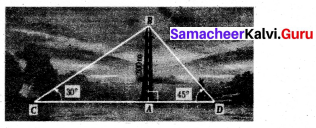$$\frac{1}{\sqrt{3}}=\frac{200}{\mathrm{AC}}$$ gives AC = 200√3
In right triangle BAD, tan 45° = $$\frac{A B}{A D}[latex] 1 = [latex]\frac{200}{\mathrm{AD}}$$ gives AD = 200
Now, CD = AC + AD = 200√3 + 200 [by (1) and (2)]
CD = 200 (√3 + 1) = 200 × 2.732 = 546.4
Distance between two ships is 546.4 m

Question 38.
Find the number of coins, 1.5 cm in diameter and 2 mm thick, to be melted to form a right circular cylinder of height 10 cm and diameter 4.5 cm.
Radius of the cylinder = $$\frac{4.5}{2} \mathrm{cm}$$
Height of the cylinder = 10 cm
Volume of the cylinder = π r2 h cu. units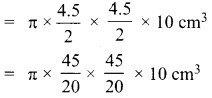Radius of the coin (r) .= $$\frac{1.5}{2} \mathrm{cm}$$
Thickness of the coin (h) = 2 mm = $$\frac{2}{10} \mathrm{cm}$$
Volume of one coin = π r2 h cu. units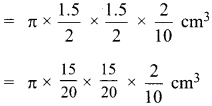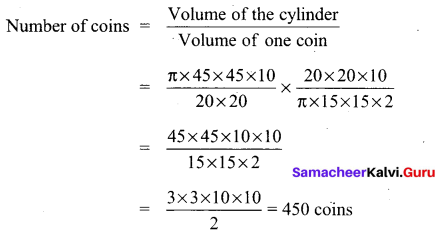Number of coins = 450Question 39.
A box contains cards numbered 3, 5, 7, 9, … 35, 37. A card is drawn at random from the box. Find the probability that the drawn card have either multiples of 7 or a prime number.
Sample space = {3, 5, 7,9 ,… ,35, 37} $$\left[\frac{37-1}{2}=\frac{36}{2}=18\right]$$
n (S) = 18
Let A be the event of getting a multiple of 7
A = {7,21,35}
n(A) = 3
p(A) = $$\frac{n(\mathrm{A})}{n(\mathrm{S})}=\frac{3}{18}$$
‘ Let B be the event of getting a prime number
B = {3,5, 7, 11, 13, 17, 19, 23.29,31.37}
n (B) = 11
p(B) = $$\frac{n(\mathrm{B})}{n(\mathrm{S})}=\frac{11}{18}$$
A∩B = {7}
n(A ∩ B) = 1
P(A ∩ B) = $$\frac{n(\mathrm{A} \cap \mathrm{B})}{n(\mathrm{S})}=\frac{1}{18}$$
p(A∪B) = P(A) + P(B) – P(A ∩ B)
$$=\frac{3}{18}+\frac{11}{18}-\frac{1}{18}=\frac{3+11-1}{18}=\frac{13}{18}$$
Probability of getting a muliple of 7 or a priime number = $$\frac{13}{18}$$

Question 40.
Find X and Y if X – Y = $$\left[ \begin{matrix} 2 & 1 \\ 4 & 3 \\ 0 & 6 \end{matrix} \right]$$ and X + Y = $$\left[ \begin{matrix} 12 & 13 \\ 6 & 5 \\ 4 & 8 \end{matrix} \right]$$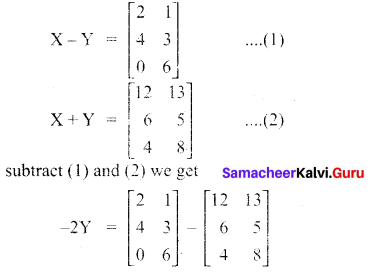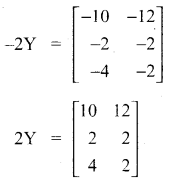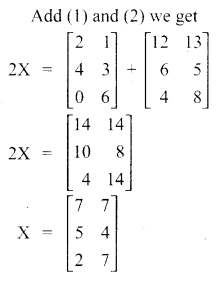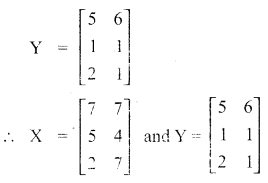Question 41.
A lead pencil is in the shape of a right circular cylinder. The pencil is 28 cm long and its radius is 3 in. If the led is of radius one mm (1 mm) then find the volume of the wood used in the pencil.
Length of a pencil (h) = 28 cm
Radius of a pencil (R) = 3mm $$\left(\frac{3}{10} \mathrm{cm}\right)$$
Radius of a led (r) = 1 mm $$\left(\frac{1}{10} \mathrm{cm}\right)$$
Volume of the wood used = Volume of the pencil – Volume of the led
= πh(R2 – r2cu. unit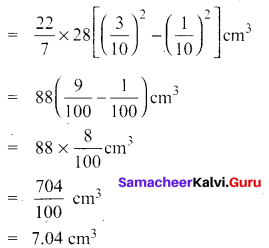Volume of the wood used in the pencil = 7.04 cm3

Question 42.
The following table shows the marks obtained by 48 students in a quiz competition in Mathematics. Calculate the variance and standard deviation.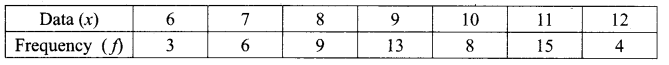Let us form the following table using the given data.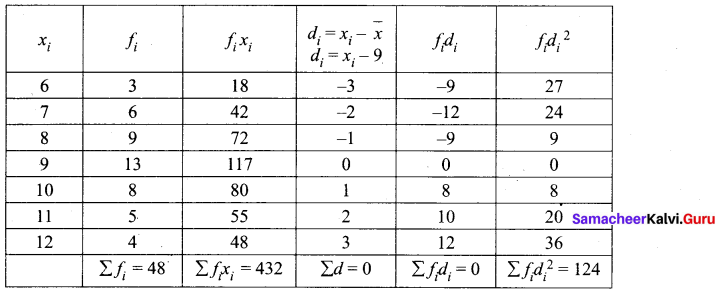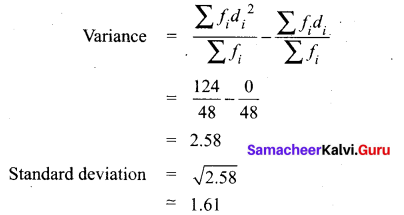PART – IV

IV. Answer all the questions. [2 × 8 = 16]

Question 43.
(a) Draw a circle of radius 4.5 cm. Take a point on the circle. Draw the tangent at that point using the alternate segment theorem. Rough Diagram
Radius of the circle = 4.5 cm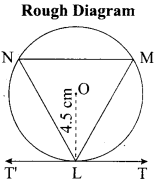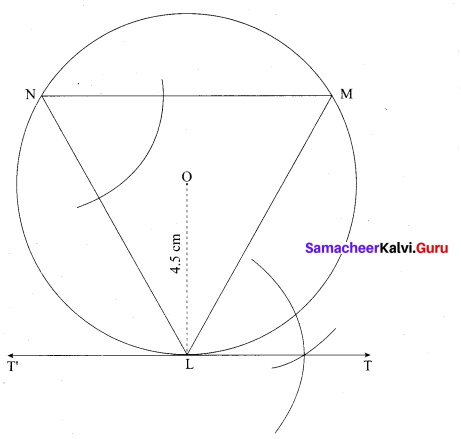Steps of construction:

1. With O as centre, draw a circle of radius 4.5 cm.
2. Take a point L on the circle. Through L draw any chord LM.
3. Take a point M distinct from L and N on the circle, so that L, M, N are in anti-clockwise direction. Join LN and NM.
4. Through “L” draw tangent TT’ such that ∠TLM = ∠MNL.
5. TT’ is the required tangent.[OR]

(b)
Construct a ∆PQR in which QR = 5 cm, ∠P = 40° and the median PG from P to QR is 4.4 cm. Find the length of the altitude from P to QR.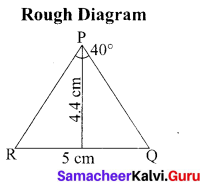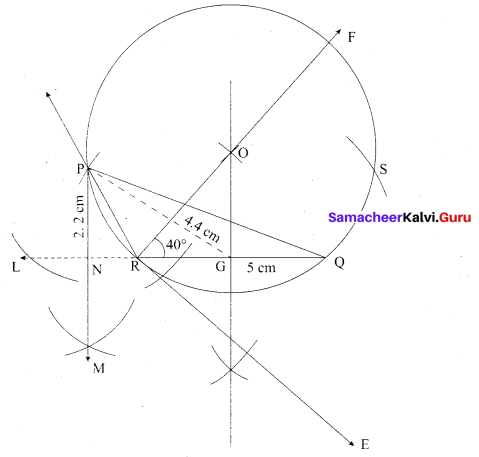Steps of construction

1. Draw a line segment RQ = 5 cm.
2. At R draw RE such that ∠QRE = 40°.
3. At R. draw RF such that ∠ERF = 90°.
4. Draw the perpendicular bisector to RQ, which intersects RF at O and RQ at G.
5. With O as centre and OP as radius draw a circle.
6. From G mark arcs of radius 4.4 cm on the circle. Mark them as P and S.
7. Join PR and PQ. Then ∆PQR is the required triangle.
8. From P draw’ a line PN which is perpendicular to RQ it meets at N.
9. Measure the altitude PN.
PN = 2.2 cm.Question 44.
(a) Draw the graph of y = x2 – 4x + 3 and use it to solve x2 – 6x + 9 = 0.
Step 1: Draw the graph of y = x2 – 4x + 3 by preparing the table of values as below.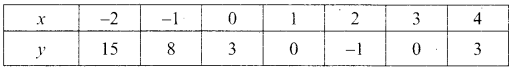Step 2: To solve x2 – 6x + 9 = 0, subtract x2 – 6x + 9 = 0 from y = – x2 – 4x + 3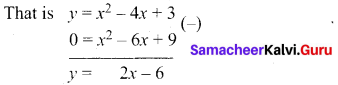The equation y = 2x – 6 represent a straight line. Draw the graph of y = 2x – 6 form in the table of values as below.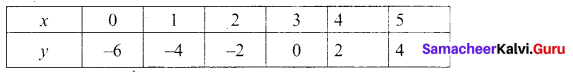The line y = 2x – 6 intersect y = x2 – 4x + 3 only at one point.

Step 3: Mark the point of intersection of the curve y = x2 – 4.r 3 and y = 2x – 6 that is (3,0).
Therefore, the x coordinate 3 is the only solution for the equation x2 – 6x + 9 = 0.[OR](b) Draw the Graph of y = x2 – 4x + 4
Let y = x2 – 4x + 4
(i) Prepare the table of values for y = x2 – 4x + 4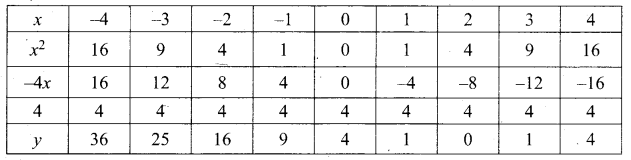(ii) Plot the points (-3, 25) (-2, 16) (-1, 9) (0, 4) (1, 1) (2, 0), (3, 1) and (4, 4)
(iii) Join the points by a free hand smooth curve.
(iv) The roots of the equation are the X-coordinates of the intersecting points of the curve with X-axis (2, 0) which is 2.
(v) Since there is only one point of intersection with X-axis (2, 0).
The solution set is 2.
∴ The Quadratic equation x2 – 4x + 4 = 0 has real and equal roots. 1 English Medium – 33″ width=”573″ height=”72″ />
The line y = 2x – 6 intersect y = x2 – 4x + 3 only at one point.

Step 3: Mark the point of intersection of the curve y = x2 – 4.r 3 and y = 2x – 6 that is (3,0).
Therefore, the x coordinate 3 is the only solution for the equation x2 – 6x + 9 = 0.